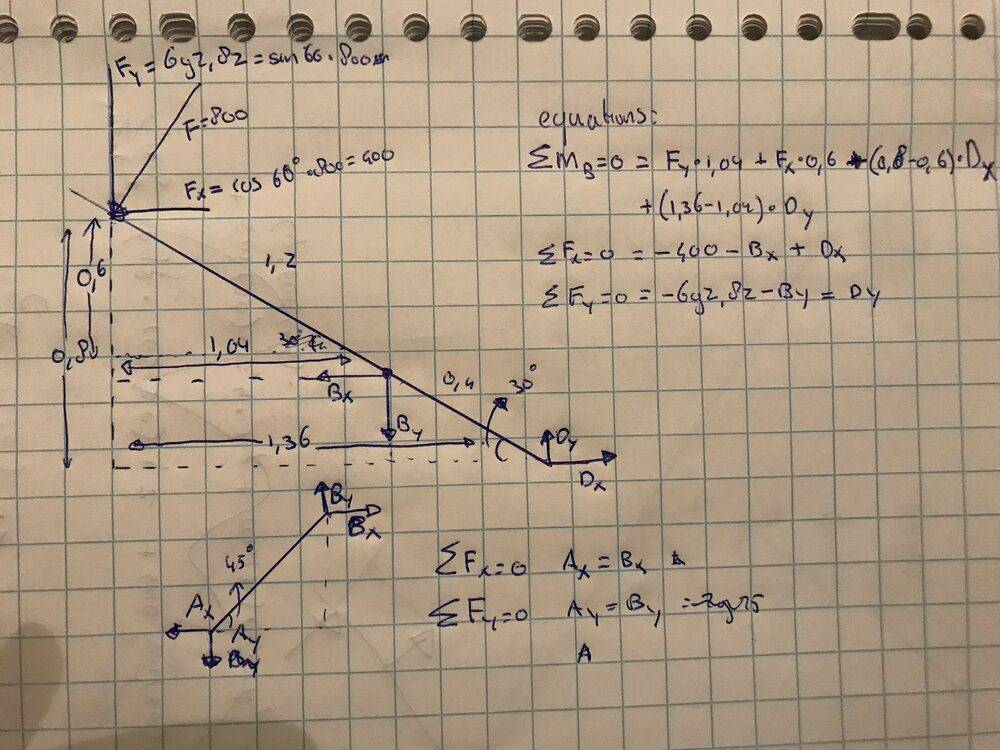# Pressure generated by a force on a pump with a lever

BiostudentWouter
Homework Statement:
Find the force in point D, both Dx and Dy
Relevant Equations:
Sum of forces = 0, sum of moment= 0#### Attachments

Last edited by a moderator:

Homework Helper
Gold Member
2022 Award
Images are for textbook extracts and diagrams. Please don't post handwritten text as images; they can be hard to read and the reader cannot cut and paste to make comments.
You have a sign error in the third equation in the top right group. Also, I couldn't read some of it. Looks like ##-6yz,\delta z##.

You need a moments equation for the short spar.

Homework Helper
Gold Member
Homework Statement:: Find the force in point D, both Dx and Dy
...
What would you like to be helped with in this problem?

Since there is resistance to compression from the fluid in cavity C, you can consider D to be an anchor to ground in order to determine instantaneous forces and torques for that particular geometry of the mechanism.

Note that the angle of 75° formed between both levers is key in calculating Dx.

Last edited: